# Python Program to Print Diamond Pattern

• Print diamond pattern of stars (*)
• Print diamond pattern of * upto n rows
• Print diamond pattern of numbers
• Print diamond pattern of alphabet characters
• Print diamond pattern of given character

## Print Diamond Pattern of Stars (*)

The question is, write a Python program to print diamond pattern using * (stars). The program given below is answer to this one of the famous question:

```rowNum = 5
space = rowNum-1
for i in range(1, rowNum+1):
for j in range(1, space+1):
print(end=" ")
space = space-1
for j in range(2*i-1):
print(end="*")
print()
space = 1
for i in range(1, rowNum):
for j in range(1, space+1):
print(end=" ")
space = space+1
for j in range(1, 2*(rowNum-i)):
print(end="*")
print()```

Here is its sample output: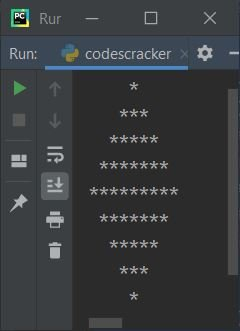## Print Diamond Pattern of * upto n Rows

This is the modified version of previous program. This program allows user to enter the number of rows or lines to print diamond pattern that expands upto given lines like shown in the program and its sample run given here:

```print(end="Enter Row Size (Total Lines): ")
rowSize = int(input())
if rowSize%2==0:
halfDiamRow = int(rowSize/2)
else:
halfDiamRow = int(rowSize/2)+1
space = halfDiamRow-1
for i in range(1, halfDiamRow+1):
for j in range(1, space+1):
print(end=" ")
space = space-1
for j in range(2*i-1):
print(end="*")
print()
space = 1
for i in range(1, halfDiamRow):
for j in range(1, space+1):
print(end=" ")
space = space+1
for j in range(1, 2*(halfDiamRow-i)):
print(end="*")
print()```

Here is its sample run with user input, 9. Since 9 is the number entered by user. Then the output will be a diamond pattern of 9 lines or rows like shown in the snapshot of sample output given below: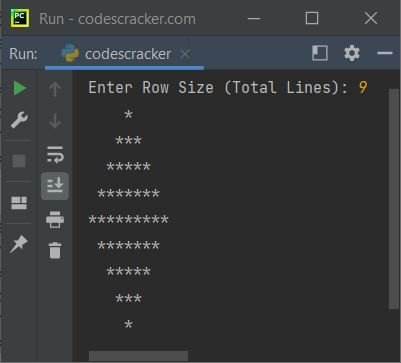## Print Diamond Pattern of Numbers

This program is similar to previous program. The only difference is, this program uses natural numbers to print diamond pattern upto given rows:

```print(end="Enter Row Size (Total Lines): ")
rowSize = int(input())
if rowSize%2==0:
halfDiamRow = int(rowSize/2)
else:
halfDiamRow = int(rowSize/2)+1
space = halfDiamRow-1
for i in range(1, halfDiamRow+1):
for j in range(1, space+1):
print(end=" ")
space = space-1
num = 1
for j in range(2*i-1):
print(end=str(num))
num = num+1
print()
space = 1
for i in range(1, halfDiamRow):
for j in range(1, space+1):
print(end=" ")
space = space+1
num = 1
for j in range(1, 2*(halfDiamRow-i)):
print(end=str(num))
num = num+1
print()```

Here is its sample run with same user input (9) as of previous program: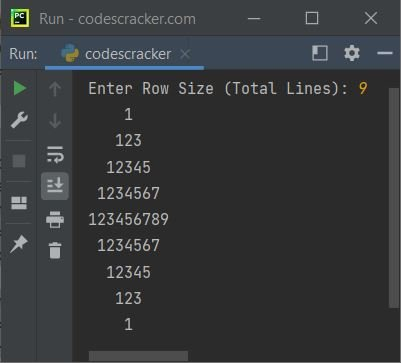## Print Diamond Pattern of Alphabet Characters

This program also prints the diamond pattern, but using alphabet characters that are A, B, C, D, etc. We've used ASCII value of first alphabet character. That is the ASCII value of A is 65. And later we've used chr() that converts ASCII value to its respective character. Let's have a look at the program and its sample run for clear understanding about all the things going on:

```print(end="Enter Row Size (Total Lines): ")
rowSize = int(input())
if rowSize%2==0:
halfDiamRow = int(rowSize/2)
else:
halfDiamRow = int(rowSize/2)+1
space = halfDiamRow-1
for i in range(1, halfDiamRow+1):
for j in range(1, space+1):
print(end=" ")
space = space-1
ascVal = 65
for j in range(2*i-1):
ch = chr(ascVal)
print(end=ch)
ascVal = ascVal+1
print()
space = 1
for i in range(1, halfDiamRow):
for j in range(1, space+1):
print(end=" ")
space = space+1
ascVal = 65
for j in range(1, 2*(halfDiamRow-i)):
ch = chr(ascVal)
print(end=ch)
ascVal = ascVal+1
print()```

Here is its sample run with again same user input, that is 9 as of previous two programs: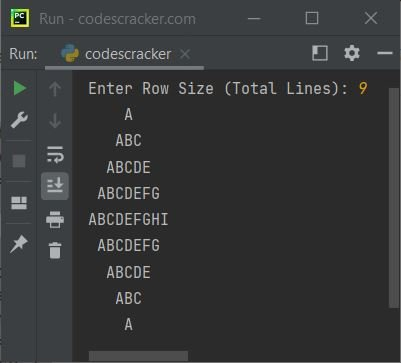## Print Diamond Pattern of Given Character

This is the last program of diamond pattern printing program. This program prints diamond pattern using any particular character, entered by user at run-time:

```print(end="Enter Row Size (Total Lines): ")
rowSize = int(input())
if rowSize%2==0:
halfDiamRow = int(rowSize/2)
else:
halfDiamRow = int(rowSize/2)+1

print(end="Enter a Character: ")
ch = input()
chLen = len(ch)
if chLen==1:
space = halfDiamRow-1
for i in range(1, halfDiamRow+1):
for j in range(1, space+1):
print(end=" ")
space = space-1
for j in range(2*i-1):
print(end=ch)
print()
space = 1
for i in range(1, halfDiamRow):
for j in range(1, space+1):
print(end=" ")
space = space+1
for j in range(1, 2*(halfDiamRow-i)):
print(end=ch)
print()
else:
print("\nOnly single character is allowed!")```

Here is its sample run with user input, 9 as row size and + as character: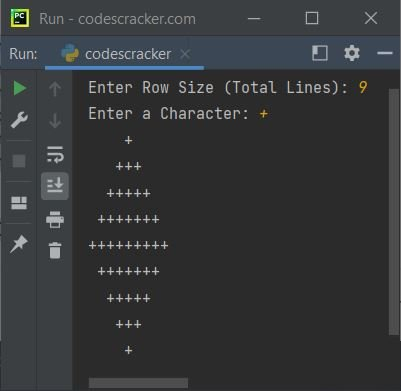Python Online Test

« Previous Program Next Program »